### Home > PC > Chapter 1 > Lesson 1.3.2 > Problem1-115

1-115.
1. Given f(x) = x2 + 1, g(x) = 2x − 8, and h(x) =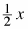+ 1. Find the following: Homework Help ✎

1. f(x) + g(x)

2. g(x)h(x)

3. g(h(x))

4. f(f(x))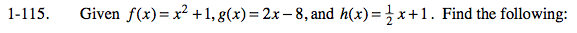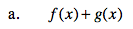$x^2+2x-7$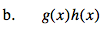$x^2-2x-8$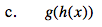$x-6$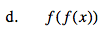$x^4+2x^2+2$